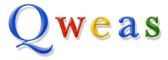bookmark this page - make qweas your homepage Help Center - What's New - Newsletter - Press Get Buttons - Link to Us - Feedback - Contact Us
 Home | Download | Top100 | Store | New Releases | Popular | Software Guides | Special Prices | Rate | Updated | FAQ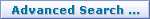All Downloads     Qweas Downloads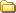Audio & MP3Video & DVDGraphics ToolsSecurity & Anti-VirusInternet UtilitiesSystem ToolsFile ConvertersMakers & DesignersBusiness Finance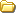Home & Education
 Calendars & Planners E-books & Literature Food & Beverage Genealogy Health & Nutrition Hobbies Home Inventory Kids & Parenting Language Mathematics Miscellaneous Music Religion & Spirituality Science Sports Teaching ToolsWeb AuthoringGame DownloadsScreensaversPocket Devices

 Web qweas.com

## Mathematics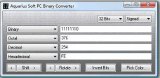Aquarius Soft PC Binary Converter - It is a simple and fast number system conversion software that lets you instantly convert between binary, octal, decimal and hexadecimal number systems. Download 1 |  Download 2 |  Buy Now |  Screenshot 1,033 KB    Windows 98, 2K, XP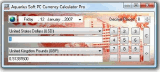Aquarius Soft PC Currency Calculator Pro - Aquarius Soft PC Currency Calculator offers the convenience to perform money convertion / exchange rate on your desktop, saving you the hassle of connecting to the Internet! Download 1 |  Download 2 |  Buy Now |  Screenshot 1,184 KB    Windows 98, 2K, XP Visual Fractal - an interesting grapher to create a graph of fractal, and graphs of Mandelbrot set and Julia set. Complex Grapher - a graphing calculator to create 3D graphs of complex function. Function Grapher Standard Edition 2.3 - Use Function Grapher to create 2D, 2.5D and 3D coordinate graphs, animations and table graphs. Geometric Constructions 1.03se - This software have 142 animations, each provides detailed description of a straightedge-and-compass geometric construction in high school geometry courses. Beautiful Calculator v3.26 - A handy replacement for the standard Windows calculator with many additional features, performming basic arithmetic, such as addition and subtraction. Breaktru Percent 3.1 - Breaktru Percent is used to calculate the percentage of any number out of any number. It is a great little tool to have on anyone's desktop. Breaktru Percent CE 2.1 - Breaktru Percent CE is used to calculate the percentage of any number out of any number. It is a great little tool to have on the Pocket PC. CalcSupreme 1.31 - Calculational software providing comprehensive basic, financial/business, math/science/engineering, and logic functions, with separate screen for each category. FRACTIONS N DECIMALS 6.3 - FRACTIONS N DECIMALS is a helpful mathematics tool that is great for school and work. It can be used to do Add, Subtract, Divide and Multiply fractions... Grab It! XP - Grab It! is a software program for getting digitized data from images. It lets you use Excel to include a photograph or chart, for example, and determine measurements or data. Grin 3.0 - Grin is a powerful tool in the Graph theory with many capabilities. Grin is easy to use; you don`t need any computer experience to get start. Inverse Matrices 1.03 - The program provides detailed, step-by-step solution in a tutorial-like format to the following problem: Given a 2x2 matrix...a 5x5 matrix. Linear Algebra - It is designed for computations of matrices. It can solution of linear systems of equations, matrix operations, finding the determinant, trace, inverse, adjoint, QR and LU factors... Linear Systems 1.02 - The program gives a process, step-by-step solution of the following problem: Given a 2x2 linear system or 2x3, or 3x2, or 3x3, or 3x4, or 4x3, or 4x4 linear system. Quick Conversion 6.6 - Quick Conversion is a piece of useful mathematics software that helps you convert units of length, weight and capacity conveniently. Random Number Generator Pro v1.34 - Generate random number. User can exclude digits from generated random number and choose lower and upper limits and increments of the numbers. Systems of Nonlinear Equations - Systems of Nonlinear Equations numerically solves systems of simultaneous nonlinear equations. Calculator Prompter 2.2 - Calculator Prompter is a math expression calculator. You can evaluate expressions like sin(cos(tan(pi)))#2%10^3 . BREAKTRU FRACTIONS N DECIMALS 6.3 - Two programs in one. One that can Add, Subtract, Divide and Multiply fractions and another that can convert a decimal to a fraction or a fraction to a decimal. Sicyon Lite calculator 4.0 - Sicyon = scientific (VBScript/JScript) calculator + matrix operations + constant database + units converter + solver + curve-fit. Mental Math Essential 2.2 - Mental Math Essential is a mental math training software focusing on mental math of addition, subtraction, multiplication and division. Conversions+ 2.0.2 - Easily convert linear, area, temperature, weight, liquid, volume, speed, date and time measurements. FindGraph 1.571 - Mental Math Essential is a mental math training software focusing on mental math of addition, subtraction, multiplication and division, neural networks... Quick Conversion 6.6 - It is a piece of useful mathematics software that helps you convert units of length, weight and capacity conveniently. Random Number Generator Pro v1.34 - Random Number Generator Pro is a Windows based application designed to generate random numbers. Sicyon Lite calculator 4.0 - Sicyon = scientific (VBScript/JScript) calculator + matrix operations + constant database + units converter + solver + curve-fit. Systems of Nonlinear Equations 1.0 - Systems of Nonlinear Equations is a software program for solving systems of simultaneous nonlinear equations. Karnaugh Analyzer 1.0 - Not only analyze Karnaugh Map, but also input your data in many form (Karnaugh Map, True Table, Function form - Boolean algebra, and in set's of Term) Math Flight 2.2 - Children can now have fun while learning and practicing basic math! Soar high into the sky in "Flight!", watch parcels being dropped by parachutes and catch the correct ones in "Drop Zone". AVD Volume Calculator 5.3.1 - AVD Volume Calculator is a handy tool for calculating weight, volume and mass of various composite objects in three dimensions.